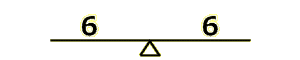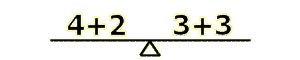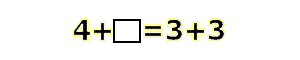# The Very Basics of Algebra

### Introduction to Algebra

Algebra sounds like a difficult concept, and you may have heard that it is from an older sibling or parent. Don't worry-- it's not hard at all. The basic idea is that we have an equation that is balanced on both sides. Picture a scale in your head with the same values on each side, and you have a basic understanding of algebra.Notice how there is a six on each side, and so they are equal and the scale is balanced? That is your first equation! You've probably realized too that we can perform operations on each side of the equation (scale). Writing 4+2 on one side and 3+3 on the other will keep the scale balanced, right?Of course, you don't have to use addition. Any of the other arithmetic operations, like subtraction, multiplication, or division will work, so long as each side is equal to the other. We will now convert the picture of the scale into an equation. This is a simple process, and all you have to do is place your two values on opposite sides of an equals sign.That's a great equation we have created, but it serves no purpose. It is obvious to anyone that 4+2=3+3, because 6=6. The equation is useful in finding the value of a missing number. Say we had a equation like this:The empty box indicates a missing number. We know something should go there, but what? This equation can be solved by adding 3+3 to get 6, and then recognizing that 4+2=6. The number 2 is the missing number. Now we have actually accomplished something with our new friend, the equation.

The next step in algebra is learning about a variable. Variables are defined as numbers that can change value or represent a missing value. The empty box in our last equation was a variable, but drawing cute little shapes can get tiresome. Variables are usually represented by letters of the alphabet, and for whatever reason x, y, and z are the most commonly used variables. Here's how our last equation would look using a variable:

$$4+x=3+3$$

Notice that the 'x' is a variable, NOT the multiplication sign. It is customary in math to use the * sign to represent multiplication so that variables don't get confused with operations. Our goal in the equation is to solve for the variable x, and you might be able to do so using trial and error, but there is an easier way. Since we have a balanced equation, we can do anything to one side, but we have to do the same to the other side. For instance, we can add 1 to each side, resulting in $$4+x+1=3+3+1$$. Adding 1 to only one side would create an inequality, which is not what we want.

The best way to solve is by isolating the variable on one side of the equation, so that we can tell what value it holds by looking at the other side of the equation, as in x=4. To do this, we must get rid of the 4. Since we can do anything to both sides of the equation, why not subtract 4 from each side?

$$4-4+x=3+3-4$$

On the left side of the equation, 4-4 is obviously 0, so we can get rid of that, and 3+3=6, minus 4 is 2, so we can write 2 in place of 3+3-4.

$$\cancel{4-4}+x=3+3-4$$ $$x=6-4$$ $$x=2$$

Remember- the goal is to get the variable alone by doing the same thing to each side. In an equation like 4x + 4 = 12, you would subtract 4 from each side to get 4x = 8. Divide each side by four and you've got x=2:

$$4x+4=12$$ $$4x+4-4=12-4$$ $$4x=8$$ $$\frac{4x}{4}=\frac{8}{4}$$ $$x=2$$

Now you've got a basic understanding of algebra, and can solve equations like $$7+x=14$$. If not, you might want to re-read the lesson, browse some other algebra lessons, or ask for help.